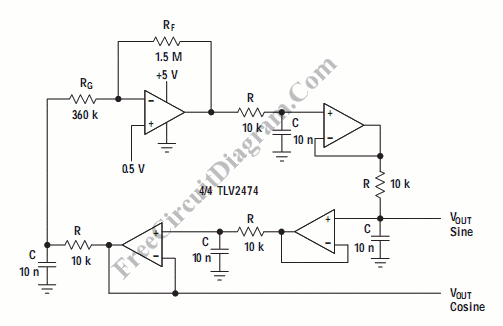# Bubba OscillatorBubba oscillator is another example of phase shift oscillator. The Bubba oscillator takes the advantage of quad op-amp package to get 45 degree phase shift for each section. The result is excellent d(phase)/d(t) to get very low frequency drift. Here is the schematic diagram of the circuit:Each RC section give 45 degree phase shift. A low impedance quadrature output is take from the alternating section. If we take the output from each op-amp, then each outputs will have 45 degrees differences. The frequency of this circuit will be about 1.76kHz. The gain is set by the RF and RG, and it should be slightly higher than 4.  The juction betwee R and RG gives the lowest distortion output. If  very low distortion is needed on all outputs, then the gain should be distributed equally on all op-amps. [Schematic diagram source:  Texas Instrument Analog Applications Journal]